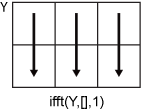# ifft

## 语法

``X = ifft(Y)``
``X = ifft(Y,n)``
``X = ifft(Y,n,dim)``
``X = ifft(___,symflag)``

## 说明

````X = ifft(Y)` 使用快速傅里叶变换算法计算 `Y` 的逆离散傅里叶变换。`X` 与 `Y` 的大小相同。 如果 `Y` 是向量，则 `ifft(Y)` 返回该向量的逆变换。如果 `Y` 是矩阵，则 `ifft(Y)` 返回该矩阵每一列的逆变换。如果 `Y` 是多维数组，则 `ifft(Y)` 将大小不等于 1 的第一个维度上的值视为向量，并返回每个向量的逆变换。 ```

``X = ifft(Y,n)` 通过用尾随零填充 `Y` 以达到长度 `n`，返回 `Y` 的 `n` 点傅里叶逆变换。`

``X = ifft(Y,n,dim)` 返回沿维度 `dim` 的傅里叶逆变换。例如，如果 `Y` 是矩阵，则 `ifft(Y,n,2)` 返回每一行的 `n` 点逆变换。`

``X = ifft(___,symflag)` 支持上述语法中的任何输入参数组合，且可指定 `Y` 的对称性。例如，`ifft(Y,'symmetric')` 将 `Y` 视为共轭对称。`

## 示例

```X = [1 2 3 4 5]; Y = fft(X)```
```Y = 1×5 complex 15.0000 + 0.0000i -2.5000 + 3.4410i -2.5000 + 0.8123i -2.5000 - 0.8123i -2.5000 - 3.4410i ```

`ifft(Y)`
```ans = 1×5 1 2 3 4 5 ```

`ifft` 函数允许您控制变换的大小。

```Y = rand(3,5); n = 8; X = ifft(Y,n,2); size(X)```
```ans = 1×2 3 8 ```

`Y = [1 2:4+eps(4) 4:-1:2]`
```Y = 1×7 1.0000 2.0000 3.0000 4.0000 4.0000 3.0000 2.0000 ```
`X = ifft(Y)`
```X = 1×7 complex 2.7143 + 0.0000i -0.7213 + 0.0000i -0.0440 - 0.0000i -0.0919 + 0.0000i -0.0919 - 0.0000i -0.0440 + 0.0000i -0.7213 - 0.0000i ```
`Xsym = ifft(Y,'symmetric')`
```Xsym = 1×7 2.7143 -0.7213 -0.0440 -0.0919 -0.0919 -0.0440 -0.7213 ```

## 输入参数

• `ifft(Y,[],1)` 返回每一列的傅里叶逆变换。• `ifft(Y,[],2)` 返回每一行的傅里叶逆变换。## 详细信息

### 向量的离散傅里叶变换

`Y = fft(X)``X = ifft(Y)` 分别实现傅里叶变换和傅里叶逆变换。对于长度为 `n``X``Y`，这些变换定义如下：

`$\begin{array}{l}Y\left(k\right)=\sum _{j=1}^{n}X\left(j\right)\text{\hspace{0.17em}}{W}_{n}^{\left(j-1\right)\text{​}\left(k-1\right)}\\ X\left(j\right)=\frac{1}{n}\sum _{k=1}^{n}Y\left(k\right)\text{\hspace{0.17em}}{W}_{n}{}^{-\left(j-1\right)\text{​}\left(k-1\right)},\end{array}$`

`${W}_{n}={e}^{\left(-2\pi i\right)/n}$`

## 算法

• `ifft` 函数测试 `Y` 中的向量是否共轭对称。如果 `Y` 中的向量共轭对称，则逆变换的计算速度更快，并且输出为实数。

如果 $g\left(a\right)={g}^{*}\left(-a\right)$，则函数 $g\left(a\right)$ 为共轭对称函数。然而，时域信号的快速傅里叶变换有一半频谱处于正频率，另一半处于负频率，第一个元素保留用于零频率。因此，当 `v(2:end)` 等于 `conj(v(end:-1:2))` 时，向量 `v` 为共轭对称向量。